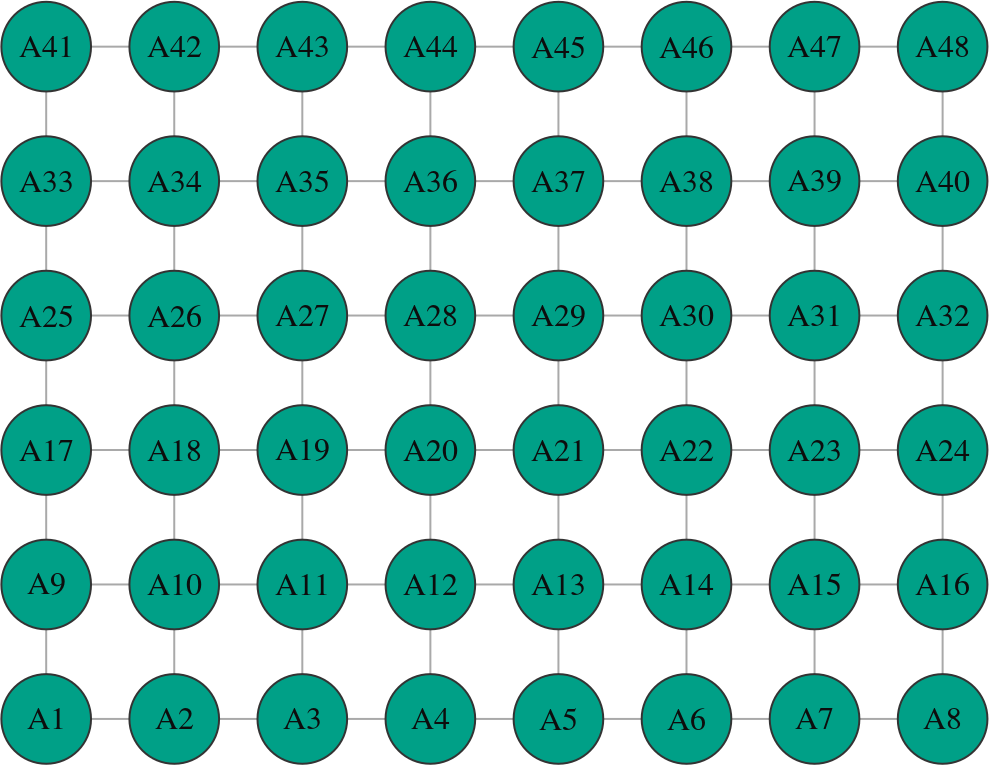# 1 Getting started

`diffuStats` is an R package providing several scores for diffusion in networks. While its original purpose lies on biological networks, its usage is not limited to that scope. In general terms, `diffuStats` builds several propagation algorithms on the package (Csardi and Nepusz 2006) classes and methods. A more detailed analysis and documentation of the implemented methods can be found in the protein function prediction vignette.

To get started, we will load a toy graph included in the package.

``````library(diffuStats)
data("graph_toy")``````

Let’s take a look in the graph:

``graph_toy``
``````## IGRAPH 9a7b9df UN-- 48 82 -- Lattice graph
## + attr: name (g/c), dimvector (g/n), nei (g/n), mutual (g/l), circular
## | (g/l), layout (g/n), asp (g/n), input_vec (g/n), input_mat (g/n),
## | output_vec (g/n), output_mat (g/n), input_list (g/x), name (v/c),
## | class (v/c), color (v/c), shape (v/c), frame.color (v/c), label.color
## | (v/c), size (v/n)
## + edges from 9a7b9df (vertex names):
##   A1 --A2  A1 --A9  A2 --A3  A2 --A10 A3 --A4  A3 --A11 A4 --A5  A4 --A12
##   A5 --A6  A5 --A13 A6 --A7  A6 --A14 A7 --A8  A7 --A15 A8 --A16 A9 --A10
##  A9 --A17 A10--A11 A10--A18 A11--A12 A11--A19 A12--A13 A12--A20 A13--A14
##  A13--A21 A14--A15 A14--A22 A15--A16 A15--A23 A16--A24 A17--A18 A17--A25
## + ... omitted several edges``````
``plot(graph_toy)``In the next section, we will be running diffusion algorithms on this tiny lattice graph.

# 2 Specifying the input

The package `diffuStats` is flexible and allows several inputs at once for a given network. The input format is, in its most general form, a list of matrices, where each matrix contains measured nodes in rows and specific scores in columns. Differents sets of scores may have different backgrounds, meaning that we can specifically tag sets of nodes as unlabelled. If we dispose of a unique list of nodes for label propagation, we should provide a list with a unique column vector that contains `1`’s in the labels in the list and `0`’s otherwise.

In this example data, the graph contains one input already.

``````input_vec <- graph_toy\$input_vec

``````##  A1  A2  A3  A4  A5  A6  A7  A8  A9 A10 A11 A12 A13 A14 A15
##   0   0   0   0   0   0   0   0   0   0   0   0   1   0   0``````

Let’s check how many nodes have values

``length(input_vec)``
``##  48``

We see that all the nodes have a measure in each of the four score sets. In practice, these score sets could be disease genes, pathways, et cetera.

# 3 The diffusion algorithm

Each one of these columns in the input can be smoothed using the network and new value will be derived - unlabelled nodes are also scored. This is the main purpose of diffusion: to derive new scores that intend to keep the same trends as the scores in the input, but taking into account the network structure. Equivalently, this can be regarded as a label propagation where positive and negative examples propagate their labels to their neighbouring nodes.

Let’s start with the simplest case of diffusion: only a vector of values is to be smoothed. Note that these values must be named and must be a subset or all of the graph nodes.

``````output_vec <- diffuStats::diffuse(
graph = graph_toy,
method = "raw",
scores = input_vec)

``````##         A1         A2         A3         A4         A5         A6         A7
## 0.03718927 0.04628679 0.04718643 0.06099494 0.09567369 0.04866964 0.02124098
##         A8         A9        A10        A11        A12        A13        A14
## 0.01081382 0.06528103 0.10077145 0.08146401 0.10111963 0.27303017 0.07776389
##        A15
## 0.02548044``````

# 4 Diffusion scores visualisation

The best way to visualise the scores is overlaying them in the original lattice. `diffuStats` also comes with basic mapping functions for graphical purposes. Let’s see an example:

``````igraph::plot.igraph(
graph_toy,
vertex.color = diffuStats::scores2colours(output_vec),
vertex.shape = diffuStats::scores2shapes(input_vec),
main = "Diffusion scores in our lattice"
)``````